# Quicksort: one of the fastest sorting algorithms!

Quick Sort is one of the most popular sorting algorithms that use the divide and conquer approach of problem-solving. Here are some excellent reasons to learn this algorithm :

• Often the best choice of sorting because it works O(nlogn) average.
• One of the best algorithms to learn the idea of recursion. Its recursive structure, the flow of recursion, and the base case are intuitive.
• An in-place sorting that works best in the virtual memory environment.
• A good algorithm to learn the worst, best, and average-case analysis.
• The Quicksort partition is an excellent idea to learn problem-solving using the two-pointers. We can use a similar approach to solve other coding questions.
• A good algorithm to understand the idea of the randomised algorithm. Randomisation is a general tool to improve efficiency of real-time systems.

### Quicksort Intuition

Let’s explore the general pattern of the sorted array and think! Suppose all the elements are arranged in increasing order.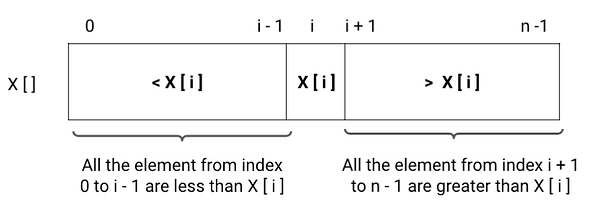From the above observation, let’s think in a reverse way: Suppose we partition an unsorted array into two parts around some value present in the array (pivot). What will happen after the partition? Here is an idea:

• All the values in the left half of the array will be less than the pivot.
• All the values in the right half of the array will be greater than the pivot.
• pivot value will get placed at the correct position in the sorted output.

Now we have a situation similar to the sorted array, but there is one difference : both left and right halves are still unsorted. If we sort both the halves, then our whole array will get sorted. Think!

If we explore closely, both unsorted halves are smaller sub-problem of the original sorting problem, i.e., a similar sorting problem for smaller input sizes. So the idea would be simple : if we sort both the halves then the whole array will be sorted because all the values in the left half are less than X[i], the pivot value is at the correct position after the partition and all the values in the right half are greater than X[i]. Think!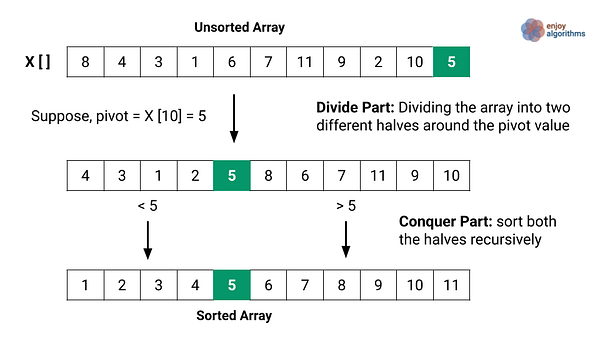The above approach is a divide and conquers approach of problem-solving : we divide the sorting problem into two smaller subproblems of sorting using the partition and solving each sub-problems recursively to get the final sorted array. At each step of the recursion, we repeat the same process of partition for the smaller sub-array.

### Divide and conquer idea of the quicksort

Divide part : We divide the problem into two smaller sub-problems by partition the array X[l…r] into two smaller subarrays around some pivot in the array. The partition process returns the pivotIndex i.e. index of the pivot in the sorted array.

• Left subproblem: Sorting array X[] from l to pivotIndex - 1
• Right subproblem : Sorting array X[] from pivotIndex + 1 to r

Conquer part : Now, we solve both the sub-problems or sort both the smaller arrays recursively. We need not worry about the solutions to the sub-problems because recursion will do the rest of the work for us.

Combine part : There is no need to combine parts because after sorting both smaller arrays, our whole array will get sorted automatically. Think!

### Divide and conquer implementation of the quicksort

Suppose the function quickSort(int X[], int l, int r) sorts the entire array where l and r are the left and right ends of the array. The initial function call would be quickSort(X, 0, n-1).

Divide part

Let’s take a partition(X, l, r) function that divides the array around a pivot and returns the pivotIndex. We have selected the pivot value inside the partition function.

``````pivotIndex = partition(X, l, r)
``````

Conquer part

• We recursively call quickSort(X, l, pivotIndex - 1) to sort the left subarray. We are passing pivotIndex - 1 as the right end of the array.
• We recursively call quickSort(X, pivotIndex + 1, r) to sort the right subarray. We are passing pivotIndex + 1 as the left end of the array.

Base case

This is the most critical step of the implementation. The base case would the smallest version of the problem where recursion terminates and returns the value directly. What would be the base case? If we find l ≥ r during the recursive calls, the sub-array would be either empty or has one value left. Our recursion should not go further and return from here.

Quicksort pseudocode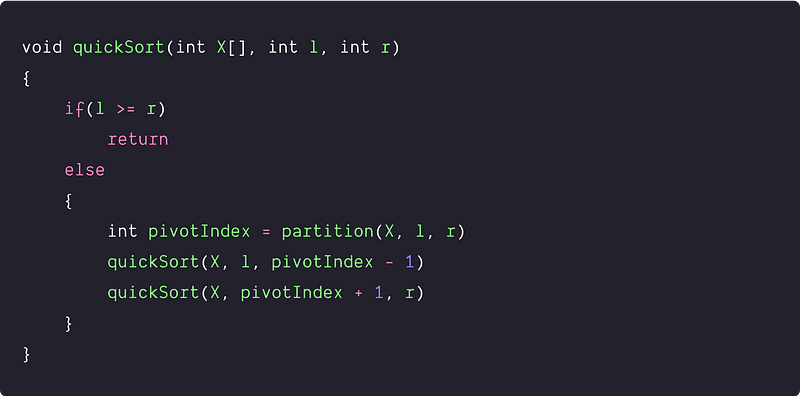### Quicksort partition algorithm

How do we divide the input array around some pivot value so that values in the left part would be less than pivot and values in the right part would be greater than pivot? Can we do it in place? Let’s think!

Suppose we pick the rightmost value as the pivot, i.e., pivot = X[r], and scan the array from left to right. Whenever we found a value less than the pivot, we place it at the starting part of the array and move forward. Otherwise, we ignore the value and move forward. The idea looks simple, but how do we implement it? Let’s think!

• We need two pointers to track two parts in the array: The current index of the array (pointer j) and the end of the starting subarray where values are less than the pivot (pointer i). Initially, we start with an empty left subarray i.e. j = l  - 1.
• Now we scan the array using a loop from j = l to r  -  1.
• Whenever we found X[i] < pivot, we increment pointer jand swap X[j] with X[i]. Otherwise, we ignore the value and move to the next iteration. Here loop will terminate when j = r.
• By the end of the loop: All the values in subarray X[0…i] will be less than the pivot, and all the values in the subarray X[i + 1 … r - 1] will be greater than the pivot.
• Now we place the pivot at its correct position i.e. index i + 1. So we swap the X[r] with X[i + 1] to complete the partition process. Think!
• Finally, we return the position of the pivot as an output i.e. return i + 1.

Pseudocode of the partition algorithm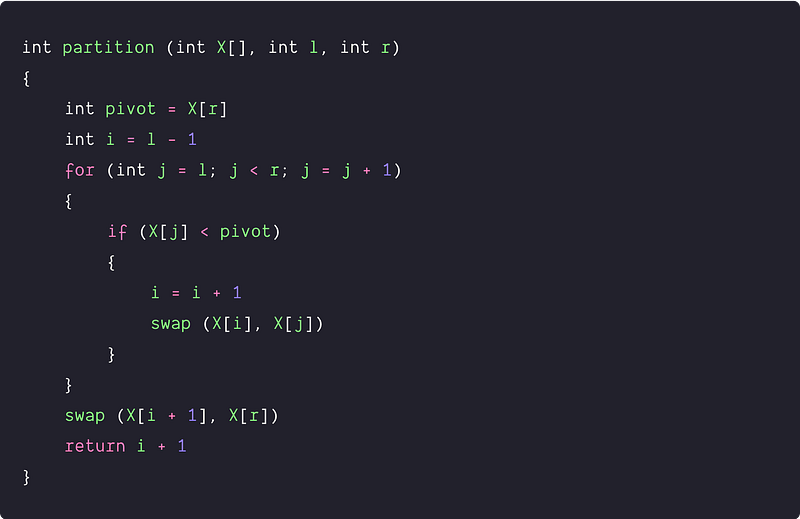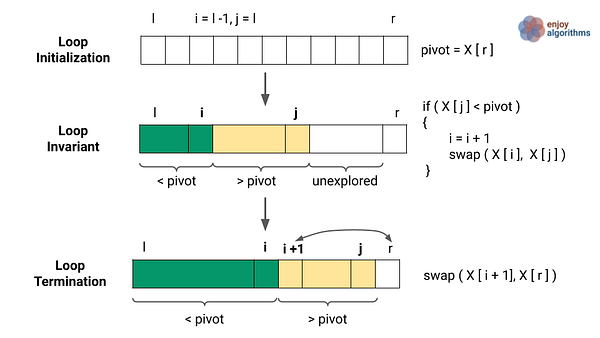Analysis of the partition algorithm

We are running a single loop and doing constant operations at each iteration. In the worst or best case, we are making one comparison at each iteration of the loop. Swapping operation depends on the comparison if (X[j] < pivot). So time complexity = O(n). We are using constant extra space, so Space complexity = O(1)

### Quicksort visualization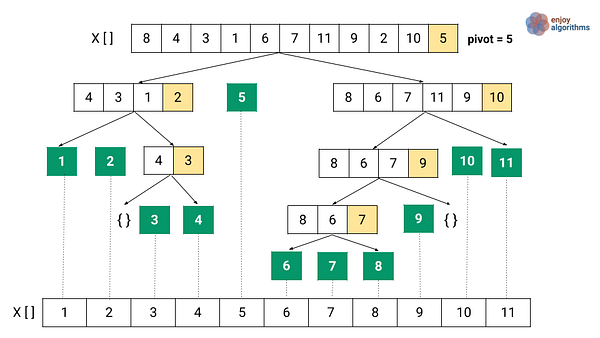### Quicksort time complexity analysis

Let’s assume that T(n) is the worst-case time complexity of quicksort for n integers. Let's analyze it by breaking down the time complexities of each  process:

Divide part: The time complexity of the divide part is equal to the time complexity of the partition algorithm, which is O(n).

Conquer part: We are recursively solving two subproblems of different sizes. The size of the subproblems depends on the choice of the pivot in the partition process! Suppose after the partition, i elements are in the left subarray (left of the pivot), and n - i - 1 elements are in the right subarray (right of the pivot).

• Size of the left subproblem = i
• Size of the right subproblem = n  -  i  -  1

Time complexity of the conquer part = Time complexity of the left sub-problem + Time complexity of the right sub-problem = T(i) + T(n - i - 1)

Combine Part: As mentioned above, there is no operation in this part of the quick sort. Time complexity = O(1).

``````For calculating the T(n), we need to add
the time complexities of the
divide, conquer, and combine part:

T(n)
= O(n) + T(i) + T(n — i — 1) + O(1)
= T(i) + T(n — i — 1) + O(n)
= T(i) + T(n — i — 1) + cn

Recurrence relation of the quick sort
T(n) = c, if n = 1
T(n) = T(i) + T(n — i — 1) + cn, if n > 1
``````

Worst-case analysis

The worst-case behavior for quicksort occurs when the partition process always picks the largest or smallest element as a pivot. In this scenario, the partition process would be highly unbalanced, i.e., one subproblem with n - 1 elements and the other with 0 elements. Such a situation will occur when the array is already sorted in increasing or decreasing order. (Think!)

Let us assume that this unbalanced partitioning arises in each recursive call. So for the time complexity in the worst case, we put i = n  - 1 in the above formula of T(n).

``````T(n)
= T(n - 1) + T(0) + cn
= T(n - 1) + cn
``````

Analysis of worst-case using the substitution method

We simply expand the recurrence relation by substituting the all intermediate value of T(i) (from i = n-1 to 1). By end of this process, we ended up in a sum of arithmetic series.

``````T(n)
= T(n-1) + cn
= T(n-2) + c(n-1) + cn
= T(n-3) + c(n-2) + c(n-1) + cn
………… and so on
= T(1) + 2c + 3c + ... + c(n-3) + c(n-2) + c(n-1) + cn
= c + 2c + 3c + ... + c(n-3) + c(n-2) + c(n-1) + cn
= c (1 + 2 + 3... + n-3 + n-2 + n-1 + n)

this is a simple sum of the arithmetic progression.
T(n) = c (n(n+1)/2) = O(n^2)

Worst case Time complexity of the quick sort  = O(n^2)
``````

Analysis of worst-case using recursion tree method

When we sum the total partitioning times for each level of recursion tree, we get => cn + c(n−1) + c(n−2) +⋯+ 2c + c ​= c (n + n−1 + n−2 + ⋯+ 2 + 1) = c(n(n+1)/2) = O(n^2)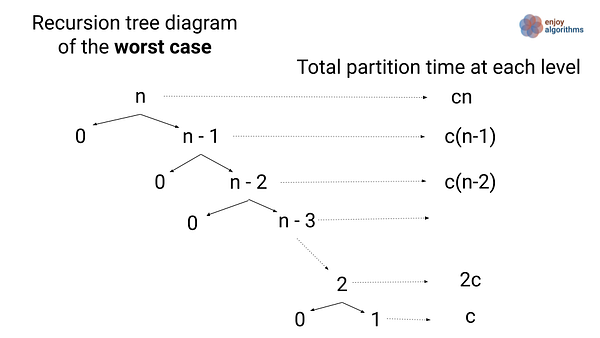Best-case analysis of quick sort

The best-case behavior for quicksort occurs when the partition process always picks the middle element as a pivot. In other words, the partition process is balanced, i.e., both sub-problems are n/2 size each. Let us assume that this balanced partitioning arises in each recursive call. So for the time complexity in the best case, we put i = n/2 in the above formula of T(n).

``````T(n) = T(n/2) + T(n - 1 - n/2) + cn
= T(n/2) + T(n/2 - 1) + cn
~ 2 T(n/2)+ cn

T(n) = 2 T(n/2)+ cn
This recurrence is similar to the merge sort for
which solution is O(nlogn).We solve this using both
recursion tree method and master theorem.

Best case Time complexity of the quick sort  = O(nlogn)
``````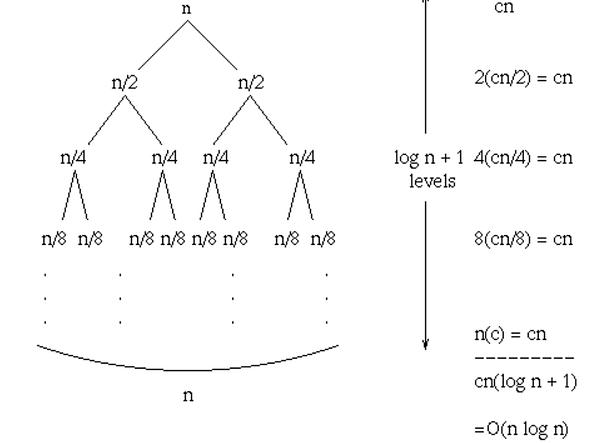Image Source —  https://www.cs.dartmouth.edu/~thc/cs5-F96/lec28.html

Average-case analysis of quicksort

The behavior of quicksort depends on the relative order of the values in the input array and not by the particular values in the array. We can assume that all permutations of the input values are equally likely. When we run quicksort on random input, the partitioning is highly unlikely to happen in the same way at every level. We expect that some of the splits will be reasonably well balanced and that some will be pretty unbalanced.

In the average case, the partition process generates a mix of good (balanced partition) and bad (unbalanced partition) splits. In a recursion tree for an average case, the good and bad splits are distributed randomly throughout the tree. Suppose, for intuition, good and bad splits appear at the alternate level of the tree.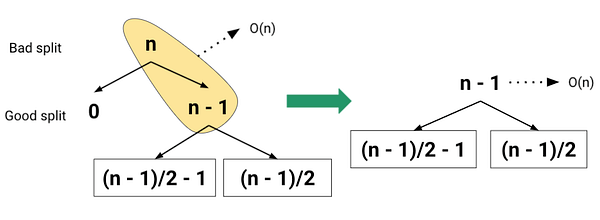Suppose at the root of the recursion tree, partition generates a good split, and at the next level, partition generates a bad split. The cost of the partition process will be O(n) at both levels. The combination of the bad split followed by the good split produces a combined partitioning cost of O(n). Certainly, this situation is equivalent to the single level of partitioning, which overall looks similar to the scenario of a balanced partition! So the average-case running time of quicksort is much closer to the best case than to the worst case. From the above perspective, the average case time complexity looks O(nlogn).

For better visualization of average-case analysis, let’s assume the partitioning algorithm always produces a partially unbalanced spit in the ratio of 9 to 1. The recurrence relation for this would be: T(n) = T(9n/10) + T(n/10) + cn. The following diagram shows the recursion tree for this recurrence . Image source: CLRS Book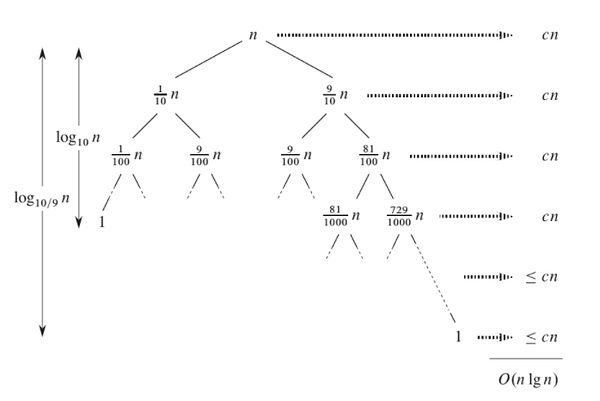We can notice the following things from the above diagram:

• The left subtree is decreasing very fast with a factor of 1/10. So the depth of the left subtree is equal to log10(n). (Think!)
• The right subtree is decreasing slowly with a factor of 9/10. So the depth of the right subtree is equal to log10/9(n). Note: log10/9(n) = O(logn)
• At every level of recursion, the cost of partition is at most cn.
• After doing the sum of cost at each level of the recursion tree, the quick sort cost is O(nlogn).
• Thus, with a 9-to-1 proportional split at every recursion level, which intuitively seems quite unbalanced, quicksort runs in O(nlogn) time. Even a 99-to-1 split can produce O(nlogn) time complexity!
• In general, any split of constant proportionality produces a recursion tree of depth O(logn), where the cost at each level is O(n). The time complexity is, therefore, O(nlogn) whenever the split has constant proportionality.

Space complexity analysis

Quicksort is an in-place sorting algorithm where we are not using additional extra space in the code. But as we know, every recursive program uses a call stack in the background to execute the recursion. So the space complexity of the quick sort depends on the size of the recursion call stack which is equal to the height of the recursion tree! (Think!) As we know from the above analysis, the height of the recursion tree depends on the partition process.

In the worst-case scenario, the partition is always unbalanced, and recursion decreases by 1 at each level. In such a scenario, the generated tree is skewed in nature, and the height of the tree = O(n). So we require a call stack of size O(n). Worst-case space complexity = O(n)

The partition is always balanced in the best-case scenario, and recursion decreases by 1/2 at each level. In such a scenario, the generated tree is balanced in nature, and the height of the tree = O(logn). So we require a call stack of size O(logn). Best case space complexity = O(logn)

### Case of repeated elements

In the above algorithm, we assume that all input values are distinct. But how do we modify the quick sort algorithm when input values are repeated? Let’s think!

In the above partition algorithm, quicksort exhibits poor performance for inputs with many repeated elements even though we choose good pivot values. To understand this better, suppose all the input elements are equal. At each stage of recursion, the left partition is empty, and the right partition has only decreased by one element. This is a situation just similar to the worst-case scenario of the quick sort. But what would be a good solution? Here is an idea!

We modify the partition algorithms and separates the input values into three groups: values less than the pivot, values equal to the pivot, values greater than the pivot. The values equal to the pivot are already sorted, so only the less-than and greater-than partitions need to be recursively sorted. In the modified partition algorithm, we return two index startIndex and endIndex such that:

• all elements of X[] from l to startIndex-1 are less than the pivot
• All elements of X[] from startIndex to endIndex are equal
• All elements of X[] from endIndex + 1 to r are greater than the pivot

Modified quicksort pseudocode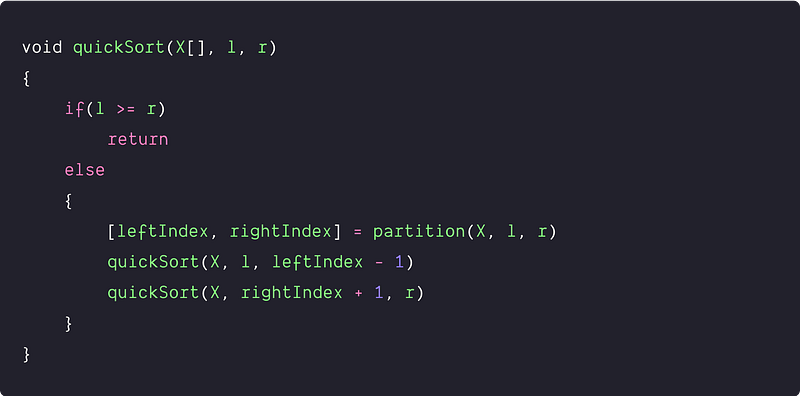### Choosing the pivot values in the partition algorithm

There are many ways to choose pivot in the quick sort.

• Always choosing the last element as a pivot.
• Always choosing the first element as a pivot.
• Selecting a random element as a pivot.
• Selecting median as a pivot.

In the above implementation, we have chosen the rightmost element as the pivot element. But this can result in a worst-case situation when an array is sorted. The best idea would be to choose a random pivot that minimizes the chance of worst-case. Selecting the median element would also be acceptable in the majority of cases. Here is the pseudo-code snippet for the median-of-three pivot selection:

``````mid = l + (r - l)/ 2
if X[mid] < X[l]
swap (X[l], X[mid])
if X[hi] < X[lo]
swap (X[l], X[r])
if X[mid] < X[r]
swap (X[mid], X[r])
pivot = X[r]
``````

### Critical ideas to think!

• What value of pivotIndex does partition return when all elements in the array X[] have the same value? What is the time complexity of Quicksort when all elements of the array have the same value?
• How would you modify Quicksort to sort into non-increasing order?
• We can improve the running time of Quicksort by taking advantage of the fast running time of insertion sort when its input is almost sorted. The idea is — after calling Quicksort on a subarray with fewer than k elements, let it simply return without sorting the subarray. After the top-level call to quicksort returns, run insertion sort on the entire array to finish the sorting process. What would be the time complexity of this algorithm? How should we pick the value of k?
• The above quicksort implementation is not stable. How can we modify the above algorithm to make it stable?
• Quicksort is an in-place sorting algorithm where we use extra space only for recursion call stack but not for manipulating the input. What would be the average case space complexity of the quick sort? How do we optimize the space complexity to O(logn) in the worst case?
• How can we implement Quicksort iteratively using stack?
• Why do we prefer Quick sort over Merge sort for sorting Arrays?
• How do we sort the linked list using the QuickSort algorithm? Why do we prefer merge sort over quicksort for sorting Linked Lists?
• The number of comparisons in Quicksort equals the number of comparisons during the construction of the BST with a sequence of insertion operations. Quicksort is also a similar version of the BST sort where both algorithms make the exact number of comparisons but in a different order.

### Content references

Enjoy learning, Enjoy coding, Enjoy algorithms!

Get well-designed application and interview centirc content on ds-algorithms, machine learning, system design and oops. Content will be delivered weekly.